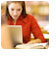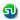### TANCET 2008 Question and solution : Linear Equations : Word Problem : Ascent Education TANCET Classes

 Home TANCET Classes GMAT Coaching Online GMAT Course CAT Classes TANCET Study Material
 TANCET '18 Classroom ProgramWeekend and weekday classes for TANCET MBA @ Chennai. At Nungambakkam and Velachery.Other Courses TANCET Correspondence Course CAT Classes & Courses GMAT Classes Chennai Online GMAT Course GRE Classes Chennai CBSE Math Online Tuition SAT Classes Other Links Ascent TANCET Toppers Testimonials CAT, TANCET Questions Careers @ Ascent Contact Us +91 44 4500 8484 +91 96000 48484 ascent@ascenteducation.com Postal Address Facebook / Twitter / Blog / Videos
You are here: Home  »  TANCET Prep Questions »  Linear Equations »   TANCET 2008 Question 1

# TANCET 2008 Quant Question 1 : Linear Equation

## Question

An owner of a pizza stand sold small slices of pizza for Rs. 150 each and large slices for Rs. 250 each. One night he sold 5000 slices, for a total of Rs. 10.50 lakh. How many small slices were sold?
1. 3000
2. 2000
3. 4000
4. 2500
5. 3500

Correct Answer : 2000. Choice (2)

This question is a word problem in linear equations. The problem is solved by framing two linear equations from the information given in the question and solving them.

Let 's' be the number of small slices and 'b' the number of large slices sold on that night.

Therefore, s + b = 5000 ... eqn (1)

Each small slice was sold for Rs.150.
Therefore, 's' small slices would have fetched Rs.150s.

Each large slice was sold for Rs.250.
Therefore, 'b' large slices would have fetched Rs.250b.

Total value of sale = 150s + 250b = 10,50,000 (note the left hand side is in Rupees and hence we have to convert right hand also to Rupees from Lakhs of Rupees)

Or 150s + 250b = 10,50,000 ... eqn (2)

Multiplying equation (1) by 150, we get 150s + 150b = 7,50,000 ... eqn (3)
Subtracting eqn (3) from eqn (2), we get 100b = 3,00,000
Or b = 3000

We know that s + b = 5000
So, s = 5000 - b = 5000 - 3000 = 2000.

2000 small slices were sold.

Level of difficulty : Easy to Moderate

## More Questions on Linear, Quadratic and Cubic Equations - Algebra

 5. 6. 7.

## CAT, XAT, TANCET Practice Questions and Answers : Listed Topicwise

 Number Theory Permutation Combination Probability Inequalities Geometry Mensuration Trigonometry Coordinate Geometry Percentages Profit Loss Ratio Proportion Mixtures Alligation Speed Time Distance Pipes Cisterns Interest Races Average, Mean AP, GP, HP Set Theory Clocks Calendars Algebra Function English Grammar General Awareness Data sufficiency TANCET Papers XAT PapersAdd to del.icio.usStumble Itdigg this